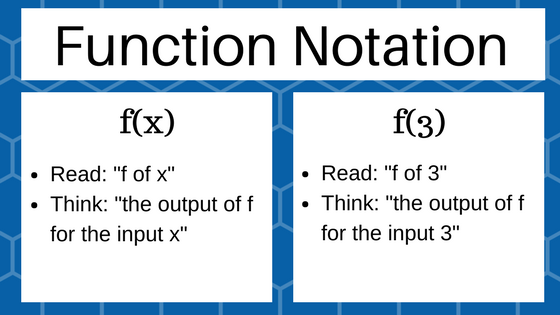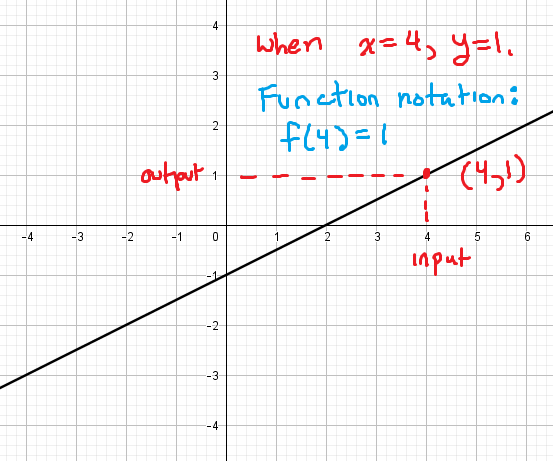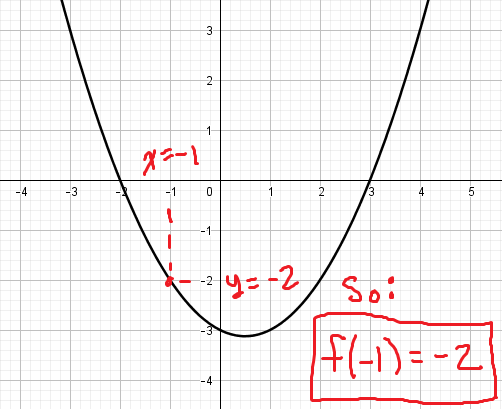﻿ Function notation and evaluating functions - MathBootCamps

# Function notation and evaluating functions

In mathematics, a function is a rule that take an input value (often $$x$$) and assigns it an output value (often $$y$$). What makes a function special is that for any given input, there is only one output. Function notation, which is used in all of mathematics, is a way of writing out the rule that relates the input and output values of a function. In this lesson, we will look at how function notation works, how to evaluate a function given the function notation, and how to evaluate a function from its graph.

Function notation is written using the name of the function and the value you want to find the output for. For example, $$f(x)$$ is read “$$f$$ of $$x$$” and means “the output of the function $$f$$ when the input is $$x$$”. Another example is something like $$g(2)$$. This is read “$$g$$ of 2” and represents the output of the function $$g$$ for the input value of 2.To help you understand this notation, let’s look at a couple of examples.

### Example

When $$x = 2$$, the value of a function $$y = f(x)$$ is 10. Use function notation to represent this.

### Solution

The function is written as $$f(x)$$. This means that the input of the function is $$x$$. Since $$x = 2$$, we can start by writing:

$$f(2)$$

The example says that when $$x = 2$$, the output is 10. Since the notation $$f(2)$$ represents the output for the input of 2, we can write this as:

$$\bbox[border: 1px solid black; padding: 2px]{f(2) = 10}$$

## Evaluating functions using function notation

When it comes to evaluating functions, you are most often given a rule for the output. To evaluate the function means to use this rule to find the output for a given input.You can do this algebraically by substituting in the value of the input (usually $$x$$). This is shown in the next couple of examples.

### Example

For $$f(x) = 2x + 1$$, find $$f(3)$$.

### Solution

$$f(x) = 2x + 1$$

Let $$x = 3$$ and then calculate the value of the function. To do this, replace each $$x$$ in the rule with 3.

$$f(3) = 2(3) + 1$$

Simplify to find the final answer.

\begin{align}&= 6 + 1\\ &= \bbox[border: 1px solid black; padding: 2px;]{7}\end{align}

When evaluating a function, make sure that you replace every $$x$$ in the rule with the input value. Pay close attention, because there may be more than one $$x$$ to replace.

### Example

For $$f(x) = 3x^2 – 5x + 1$$, find $$f(–2)$$.

$$f(x) = 3x^2 – 5x + 1$$

Let $$x = –2$$ by replacing each $$x$$ in the rule with –2 and then simplify to find your final answer.

\begin{align}f(-2) &= 3(-2)^2 – 5(-2) + 1 \\ &= 3(4) + 10 + 1\\ &= 12 + 10 + 1 \\ &= \bbox[border: 1px solid black; padding: 2px;]{23}\end{align}

It is also possible to evaluate a function using an input that is an algebraic expression. This is more complicated since you usually will need simplify your answer, but it still follows the same idea of substitution.

### Example

Let $$f(x) = –3x + 7$$. Find and simplify $$f(m + 1)$$, where $$m$$ is a real number.

### Solution

$$f(x) = -3x + 7$$

Just as you did with numbers, let $$x = m + 1$$ and evaluate the function. This means replace every $$x$$ in the rule with “$$m + 1$$”.

$$f(m+1) = -3(m+1) + 7$$

Apply the distributive property by multiplying –3 and each term within the parentheses. The combine like terms to simplify.

\begin{align}&= -3m-3 + 7\\ &= \bbox[border: 1px solid black; padding: 2px;]{-3m+4}\end{align}

From here, you can get much more complicated with things like the difference quotient, which is used later in calculus. Even with these more complicated examples, you will still apply the same concept we did to each of the examples above.

## Evaluating functions using a graph

The graph of a function is a way of viewing the outputs of the function for all possible inputs. Here, we let $$y = f(x)$$ so that each y-value represents the output and each x-value represents the input. This means any point on the graph is:

$$(x, y) = (\text{input}, \text{corresponding output})$$

Remember that in the xy-plane, the x-axis is the horizontal axis, and the y-axis is the vertical axis. Using this, we can easily read points off the graph.You can do this for the graph of just about any function. For example, if we wanted to know the value of $$f$$ when $$x = -1$$ for the function below, we would just find $$x = -1$$ on the x-axis and use the graph to find the corresponding y-value.There are some types of functions, where you have to be a little more careful. Piecewise defined functions and functions with asymptotes often have more going on in the graph. However, those are studied later on in algebra. For now, you should make sure you can read the graphs of functions like those shown in the examples above.

## Summary

Functions are used in all parts of mathematics, and understanding function notation is necessary for a wide variety of problems. Remember that it is simply a way of relating the input of a function to the value of its output. The notation $$f(x)$$ is always read “$$f$$ of $$x$$” though the function may have a different name like $$g$$ or $$k$$ (then we could write $$g(x)$$ for “$$g$$ of $$x$$” or $$k(x)$$ for “$$k$$ of $$x$$”).